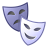# QlikView App Dev

Discussion Board for collaboration related to QlikView App Development.

Announcements
Our next Qlik Insider session will cover new key capabilities. Join us August 11th REGISTER TODAY
cancel
Showing results for
Did you mean:Contributor III

## error in expression:')' expected

Hi team,

Good dayi am facing below issue

i create two variables like below

mattend : num(sum(attend),'####0')

mnotattend  : num(sum(notattend),'####0')

using above two metrics i created variables above variables getting exact numbers but

if i use these variables in  some other calculations

like below

mtotalattend : NUM(\$(mattend) + \$(mnotattend),'###,#####')

mattend% : num(if(\$(mtotalattend)<>0,Round((\$(mattend) / \$(mtotalattend)) * 100,0.2),0),'#,##0')&'%'

i am getting error  "error in expression:')' expected"

i am not able to figure out where i did mistakeBest Regards,

Bob

9 RepliesMaster III

check that you are not using = sign before deriving variables. If you are working in qv12 version then remove extro closes of () brackets.Not applicable

Can u write = in front of your variable declaration and try again.

Also, try like this :

1.mtotalattend : NUM(\$(=mattend) + \$(=mnotattend),'###,#####')

2.mattend% : num(if(\$(=mtotalattend)<>0,Round((\$(=mattend) / \$(=mtotalattend)) * 100,0.2),0),'#,##0')&'%'

Thanks

KhushbooNot applicable

Try like below,

=num(

if(

\$(mtotalattend)<>0,

Round((\$(mattend) / \$(mtotalattend)) * 100,0.2),0)

,'#,##0%')

Make sure your variable expression should return valid result.Else it will return "error like above".MVP

Try like this

mattend : =num(sum(attend),'####0')

mnotattend  : =num(sum(notattend),'####0')MVP

Most probably, one of your \$-sign substitutions in the expressions expands to...nothing. Check spelling (lower/upper case, identical characters etc.) and proper value assignment for all variables.

If you have no idea where to start, try by elimination. Cut your expression to pieces and use each piece. Continue with the one that still generates error messages.

Best,

PeterSpecialist III

Hi Bo66y:

mattend : num(sum(attend),'####0')

mnotattend  : num(sum(notattend),'####0')

If you are formating your expression when you use the variables, you don't need to format here.

Regards!Contributor III
Author

Hi  buddys,

I tired below logic's but it not useful for me

A.

mattend : num(sum(attend),'####0')

mnotattend  : num(sum(notattend),'####0')    but again i am facing same issue

B.

1.mtotalattend : NUM(\$(=mattend) + \$(=mnotattend),'###,#####')

2.mattend% : num(if(\$(=mtotalattend)<>0,Round((\$(=mattend) / \$(=mtotalattend)) * 100,0.2),0),'#,##0')&'%'.

C.

the below logic i am getting total average but i need individual results:

like in "A class" is the dimension i need to display related to "A class" attend % but using the below code it showing total avg

mattend : =num(sum(attend),'####0')

mnotattend  : =num(sum(notattend),'####0')

i am using   qv11

Regards,

BobSpecialist III

Hy Bo66y,

Regards!Not applicable

Hi Bo66y,

- Background Color

- Text Color

- Text FormatMaybe there you can find the missing ')'

Regards,

KlausCommunity Browser